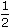# Aptitude :: Discount Mcqs-Quiz-test# 01

###### Aptitude :: Discount Mcqs-Quiz-test# 01 multiple choice question
2
Created onAptitude :: Discount

Aptitude :: Discount

1 / 10

The present worth of Rs. 2310 due 2years hence, the rate of interest being 15% per annum, is:

2 / 10

The true discount on Rs. 1760 due after a certain time at 12% per annum is Rs. 160. The time after which it is due is:

3 / 10

A man buys a watch for Rs. 1950 in cash and sells it for Rs. 2200 at a credit of 1 year. If the rate of interest is 10% per annum, the man:

4 / 10

 The true discount on a bill due 9 months hence at 16% per annum is Rs. 189. The amount of the bill is:

5 / 10

 Goods were bought for Rs. 600 and sold the same for Rs. 688.50 at a credit of 9 months and thus gaining 2% The rate of interest per annum is:

6 / 10

If Rs. 10 be allowed as true discount on a bill of Rs. 110 due at the end of a certain time, then the discount allowed on the same sum due at the end of double the time is:

7 / 10

 A man wants to sell his scooter. There are two offers, one at Rs. 12,000 cash and the other a credit of Rs. 12,880 to be paid after 8 months, money being at 18% per annum. Which is the better offer?

8 / 10

 A trader owes a merchant Rs. 10,028 due 1 year hence. The trader wants to settle the account after 3 months. If the rate of interest 12% per annum, how much cash should he pay?

9 / 10

The true discount on Rs. 2562 due 4 months hence is Rs. 122. The rate percent is:

10 / 10

 A man purchased a cow for Rs. 3000 and sold it the same day for Rs. 3600, allowing the buyer a credit of 2 years. If the rate of interest be 10% per annum, then the man has a gain of: